Next: Mathematical Operations Up: Ideal Amplifier Approximation Previous: Non-inverting Amplifiers

## Inverting Amplifiers

An inverting amplifier is shown in figure 6.4. Analysis of the circuit gives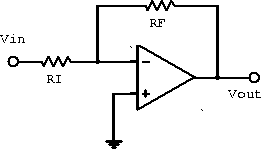Figure 6.4:  Inverting amplifier.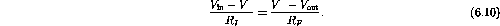Since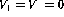(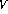is at virtual ground),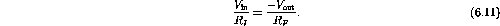The gain isThe output is inverted with respect to the input signal.

A sketch of the frequency response of the inverting and non-inverting amplifiers are shown in figure 6.5.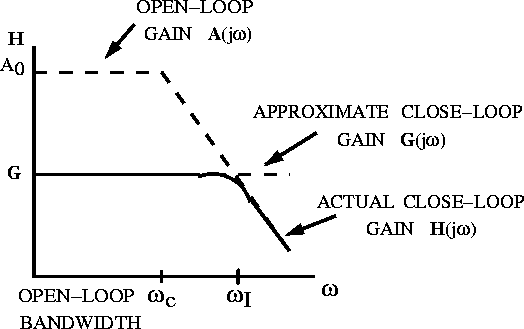Figure 6.5:  Inverting and non-inverting amplifier frequency response.

The input impedance of the inverting amplifier is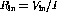. Since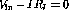we have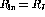.

A better circuit for approximating an ideal inverting amplifier is shown in figure 6.6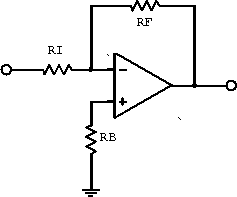Figure 6.6:  Inverting amplifier with bias compensation.

The extra resistor is a current bias-compensation resistor. It reduces the current bias by eliminating non-zero current at the inputs.

Doug Gingrich
Tue Jul 13 16:55:15 EDT 1999